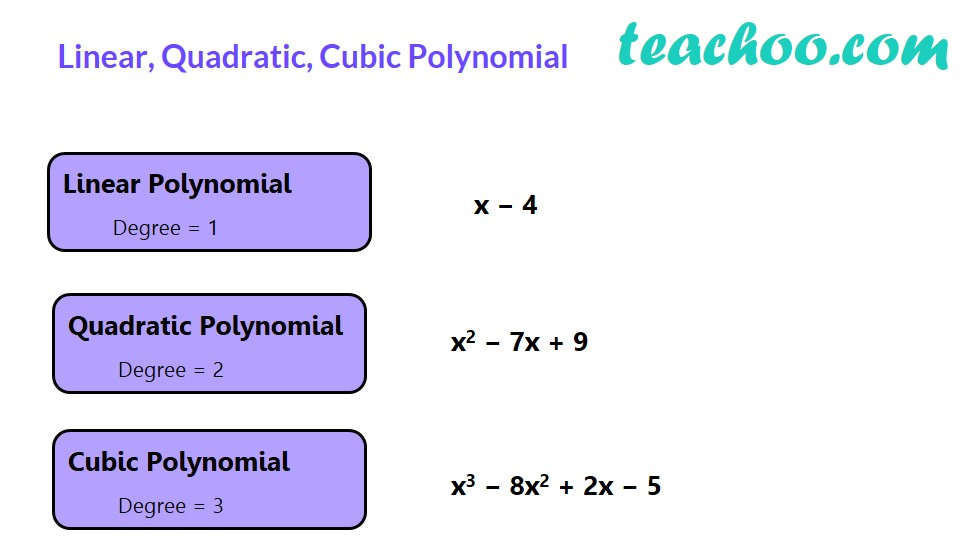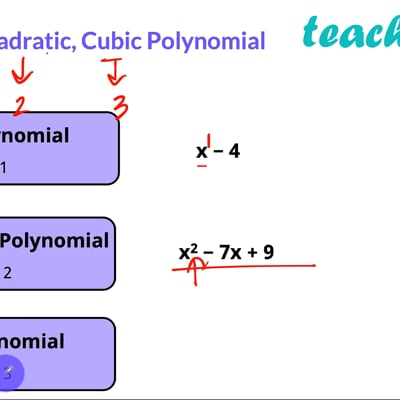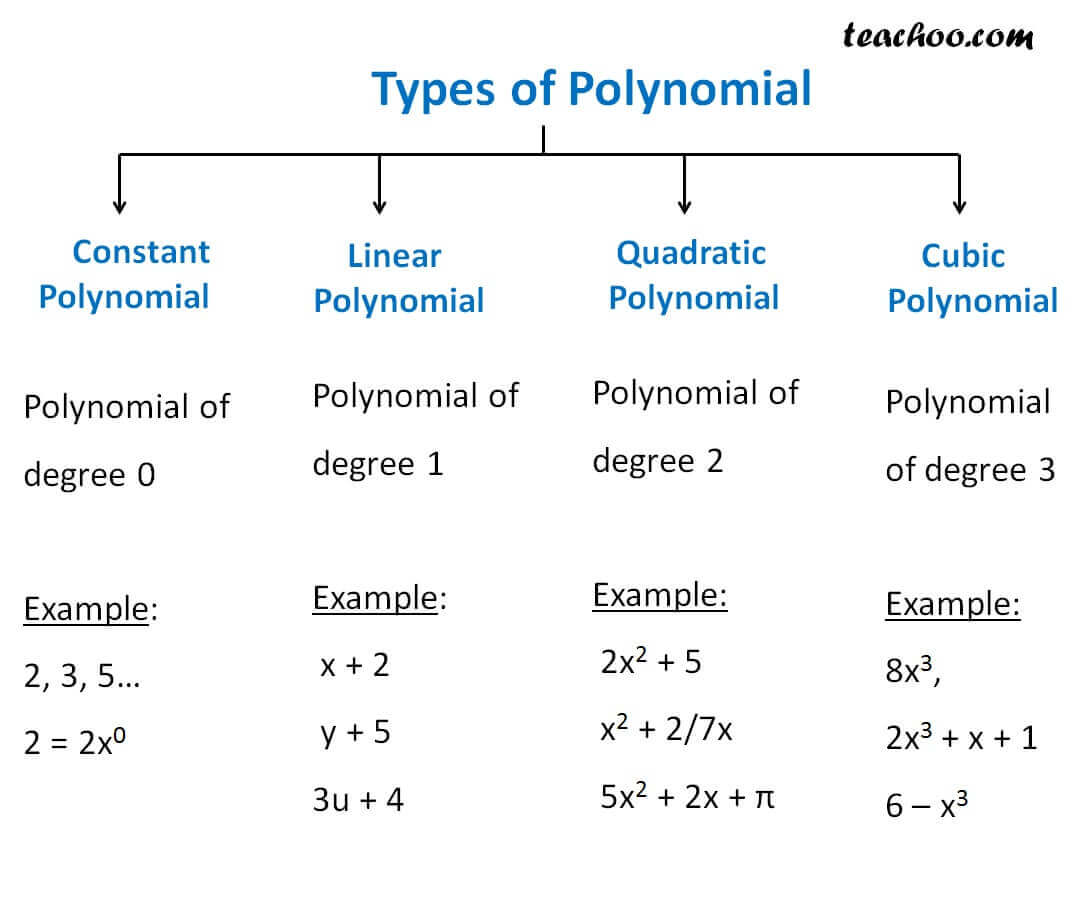Degree & Coefficient of a polynomial

Chapter 2 Class 9 Polynomials
Concept wiseThis video is only available for Teachoo black users

Polynomials are classified on degree.

If,  Degree = 3, it is cubic polynomial

Degree = 2, It is quadratic polynomial

Degree = 1, It is linear polynomial

Degree = 0, It is constant polynomial

 Degree Types of Polynomial 3 Cubic Polynomial 2 Quadratic Polynomial 1 Linear Polynomial 0 Constant PolynomialFind degree & type of polynomial

x 3 − 3x 2 + 4x + 10

-a-

Here, Degree = Highest Power = 3

So, it is  cubic polynomial.

-ea-

x 2 + 2x + 4

-a-

Degree = Highest Power = 2

-ea-

x + 30

-a-

Degree = Highest Power = 1

So, it is  linear polynomial.

-ea-

3

-a-

3 =  3 × 1

3 =  3 × 0

Degree = Highest Power = 0

So, it is  constant polynomial.

-ea-

Introducing your new favourite teacher - Teachoo Black, at only ₹83 per month

### Transcript

Linear, Quadratic, Cubic PolynomialLinear Polynomial Degree = 1 x − 4 x − 4 Quadratic Polynomial Degree = 2 x2 − 7x + 9 Cubic Polynomial Degree = 3 x3 − 8x2 + 2x − 5